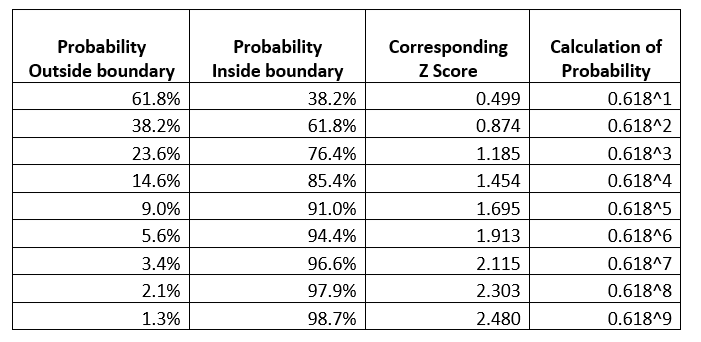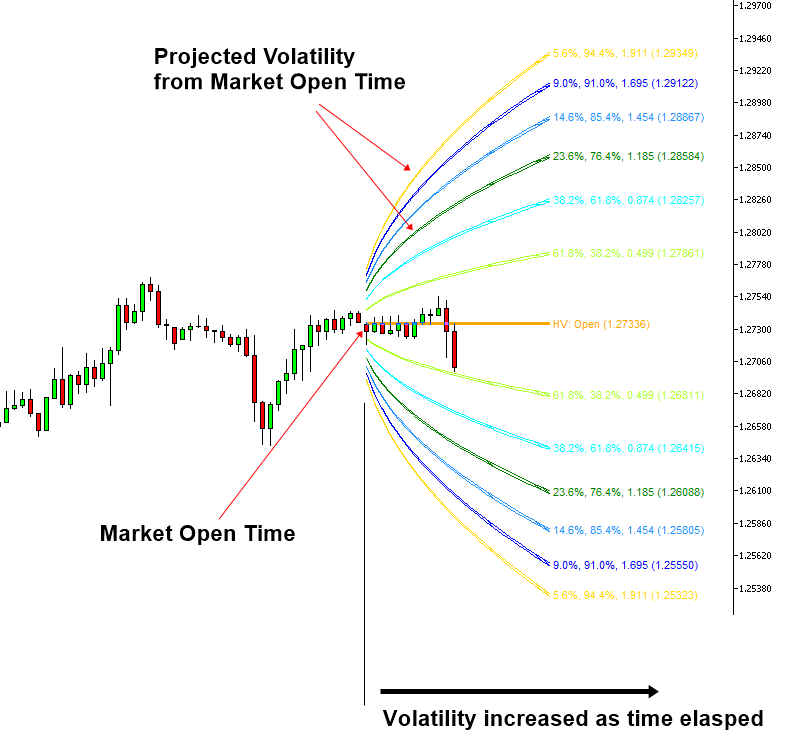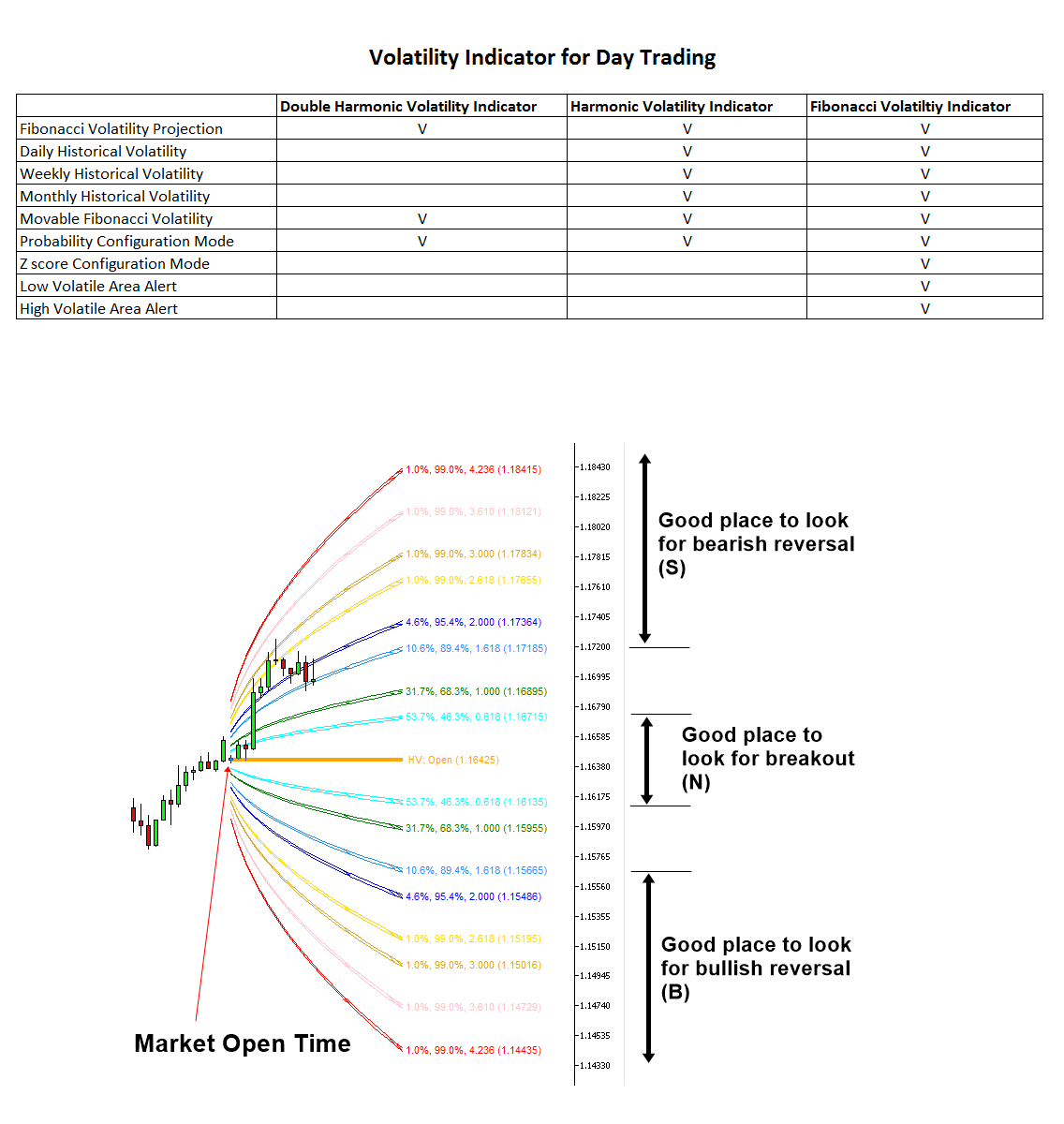# Volatility Indicator – Trading Strategies – 26 January 2022

Introduction to Volatility Index

For financial trading, volatility is widely used. It is common to see that many trading strategies are based on volatility. However, when we try to apply fluctuations in our chart, the standard deviation or variance is not very user friendly. For this reason, some traders have created a chart-friendly version of the volatility indicator. One of the many volatility tools can include the Harmonic Volatility indicator. The harmonic volatility indicator is simple yet powerful in its concept. In this chapter, we will provide a brief explanation of the Harmonic Volatility Indicator. The harmonic volatility indicator is a volatility indicator that can plot the projection of daily, weekly or monthly volatility in your chart. Plots the many probability lines that are derived from the golden ratio 0.618. Hence, it is a Fibonacci volatility indicator. The derived probability lines are fully described in Table 5.1-1. These probability lines indicate future volatility from when the market opened or from a specific point in time in your chart. They can help you adjust your entry or exit in many different ways. In this chapter, we are interested in using this volatility indicator for both reversal and breakout trading.Table 5.1-1: Derived probability using the golden ratio 0.618 for the harmonic volatility indexFigure 5.1-3: Harmonic volatility indicator on the time frame of the pair GBPUSD H1

To use the Harmonic Volatility Indicator for reversal or breakout trading, we need to identify the three volatility zones. First, the area where the probability is within the bounds within 61.8% can be considered a neutral area or a low volatility (N) area. If the price stays within this low volatility zone, we can tell that the strength of the bull and the bear is equal. The corresponding Z-score (i.e. standard deviation) is 0.874. You can check the location of this region in Figure 5.1-4. In this Neutral Zone (N), we can often catch a good breakout trading opportunity. When we want to apply more conservative criteria, you can set the neural region (N) within a 38.2% probability at a Z score of 0.499 (Fig. 5.1-5).

Secondly, a bullish reversal or potential buy zone (B) can be identified below the 61.8% probability line (Fig. 5.1-4). The corresponding Z score is 0.874. If the price is moved below this 61.8% probability line, we can assume that selling pressure can slow down. When you want to apply more conservative criteria, we can define it below the 76.4% probability line at the Z score of 1.185 (Fig. 5.1-5).

Thirdly, a bearish reversal or potential sell area (S) can be identified above the 61.8% probability line (Fig. 5.1-4). The corresponding Z score is 0.874. If the price moves above this 61.8% probability line, we can assume that the buying pressure can slow down. When you want to apply more conservative criteria, we can define it as above 76.4% probability line at Z score 1.185 (Fig. 5.1-5).

Both the bullish reversal zone (B) and the bearish reversal zone (S) are useful when you want to capture the turning point while the neutral zone (N) is useful when you want to catch a breakout trading opportunity.Figure 5.1-4: Bullish Reversal Zone (B) and Bearish Reversal Zone (S) in the Harmonic Volatility Index in a less conservative definitionFigure 5.1-5: Bullish Reversal Zone (B) and Bearish Reversal Zone (S) in the Harmonic Volatility Index in the conservative definition

Fibonacci volatility indicator

The Fibonacci volatility indicator can provide market volatility in a visual form in your chart for your trading. You can use daily, weekly, monthly and yearly fluctuations for your trading. The most important application of this volatility indicator is to spot the potential breakout area, the potential bullish reversal area, and the potential bearish reversal area. When you want to visualize volatility for your trading, this is the best tool available in the market. In addition, you can also use the moving volatility indicator.

Fibonacci Volatility Indicator For MetaTrader 4
Fibonacci Volatility Indicator For MetaTrader 5

harmonic volatility index

The Harmonic Volatility Indicator also provides market volatility in a visual form in your chart for your trading. This tool can be used to visualize daily, weekly, monthly and yearly fluctuations. The difference between harmonic volatility indicator and Fibonacci volatility indicator is that you can just use the probability configuration mode in the harmonic volatility indicator while in the Fibonacci volatility indicator you can access the probability configuration and configure the Z degree. This is cheaper than the Fibonacci volatility indicator.

Harmonic Volatility Indicator 4 for MetaTrader 4
Harmonic Volatility Indicator 4 for MetaTrader 5

double harmonic volatility indicator

This indicator is mostly specialized in moving volatility indicator. Hence, if you only need a moving volatility indicator and you want to have a more cost effective tool then use this indicator. However, if you want to have more options in visualizing volatility, use either the Harmonic Volatility indicator or the Fibonacci Volatility indicator.

Double Harmonic Volatility Indicator for MetaTrader 4

Double Harmonic Volatility Indicator for MetaTrader 5

GARCH has improved Nelder Mead (free)

This is a free volatility indicator that implements Conditional Variable Autoregressive (GARCH) methods using the Nelder Mead algorithm. This is part of the empirical indicator for achieving the GARCH(1,1) model. Hence, we provide this indicator for free. It will be interesting to see how this volatility indicator works in your trading.

GARCH has improved Nelder Mead For MetaTrader 4

GARCH has improved Nelder Mead for MetaTrader 5

Summary of the Volatility Index

We have provided a list of volatility indicator. Thus, it might be a good idea to summarize what is included in each volatility indicator. Hence, we present this screenshot.